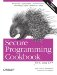# Representing a Fixed-Size Numerical Vector

Problem

You want an efficient representation for manipulating constant-sized numerical vectors

Solution

On many common software architectures, it is more efficient to use a custom vector implementation than a valarray when the size is known at compile time. Example 11-17 provides a sample implementation of a fixed-size vector template called a kvector.

Example 11-17. kvector.hpp

#include #include template class kvector { public: // public fields Value_T m[N]; // public typedefs typedef Value_T value_type; typedef Value_T* iterator; typedef const Value_T* const_iterator; typedef Value_T& reference; typedef const Value_T& const_reference; typedef size_t size_type; // shorthand for referring to kvector typedef kvector self; // member functions template void copy(Iter_T first, Iter_T last) { copy(first, last, begin( )); } iterator begin( ) { return m; } iterator end( ) { return m + N; } const_iterator begin( ) const { return m; } const_iterator end( ) const { return m + N; } reference operator[](size_type n) { return m[n]; } const_reference operator[](size_type n) const { return m[n]; } static size_type size( ) { return N; } // vector operations self& operator+=(const self& x) { for (int i=0; i

Usage of the kvector class template is demonstrated in Example 11-18.

Example 11-18. Using kvector

```#include "kvector.hpp"

#include
#include
#include

using namespace std;

int main( ) {
kvector v = { 1, 2, 3, 4 };
cout << "sum = " << accumulate(v.begin( ), v.end( ), 0) << endl;
v *= 3;
cout << "sum = " << accumulate(v.begin( ), v.end( ), 0) << endl;
v += 1;
cout << "sum = " << accumulate(v.begin( ), v.end( ), 0) << endl;
}```

The program in Example 11-18 will produce the following output:

```sum = 10
sum = 30
sum = 34```

Discussion

The kvector template in Example 11-17 is a cross between a valarray and the array template proposed for TR1. Like valarray, kvector represents a sequence of values of a given numerical type, but like TR1::array, the size is known at compile time.

Salient features of kvector are that it supports array initialization syntax and it provides begin and end member functions. In effect, a kvector is considered a pseudo-container, which means that it satisfies some but not all of the requirements of a standard container concept. The result of this is that it is much easier to use kvector with standard algorithms than a valarray.

Another advantage of the kvector template class is that it supports array initialization syntax as follows:

```int x;
kvector k = { x = 1, x + 2, 5 };```

This initializing syntax is only possible because kvector is an aggregate. An aggregate is an array or a class with no user declared constructors, no private or protected nonstatic data members, no base classes, and no virtual functions. Note that you can still declare a kvector filled with default values as follows:

`kvector k = {};`

This fills the vector with zeros.

As you can see, I had to made a design trade-off between fully satisfying the standard container requirements or allowing the array initialization syntax. A similar design trade-off was made in the design of the TR1 array template.

Perhaps the biggest advantage of the kvector over dynamic vector implementations is performance. The kvector template is much more efficient than most dynamic vector implementations for two main reasons: compilers are very good at optimizing fixed-size loops, and there are no dynamic allocations. The performance difference is particularly noticeable for small matricies (e.g., 2 2 or 3 3), which are common in many kinds of applications.

## What Is the self typedef?

The self typedef I use in Example 11-17 and in later examples is a convenient shorthand that I use to refer to the type of the current class. It makes code much easier to write and understand when the self typedef is used rather than writing out the name of the class.Secure Programming Cookbook for C and C++: Recipes for Cryptography, Authentication, Input Validation & More
ISBN: 0596003943
EAN: 2147483647
Year: 2006
Pages: 241